﻿ Exergy Analysis of a Fixed-Bed, Batch Type Rice Husk Fuelled Downdraft GasifierSustainable Energy Volume 9, 2021 - Issue 1
Publications are Open
Access in this journal
Article Versions
Export Article
• Normal Style
• MLA Style
• APA Style
• Chicago Style
Research Article
Open Access Peer-reviewed

### Exergy Analysis of a Fixed-Bed, Batch Type Rice Husk Fuelled Downdraft Gasifier

Alhaji Bukar Abubakar, Mohammed B. Oumarou , Fasiu A. Oluwole, Sahabo Abubakar
Sustainable Energy. 2021, 9(1), 9-21. DOI: 10.12691/rse-9-1-2
Received August 31, 2021; Revised September 03, 2021; Accepted September 22, 2021

### Abstract

This paper treats exergy analysis of rice husk at various loading rates of 1 kg, 1.5 kg and 2 kg of feedstock, using a batch type, fixed-bed gasifier, equipped with a gas filtering system and between the operating temperatures of 690 K and 1100 K, operated for the whole year. Exergy analysis is a thermodynamic technique which uses conservation of mass and energy principles to design thermal systems with maximum efficiency and it is based on First and Second law of thermodynamics. Formulas from literature and previous researches as well as Microsoft Excel 2007 were used to make calculations and graphically interpret the results. The exergoeconomic analysis is based on location specific and national data collected from official sources. A MATLAB based hybrid model developed from a combination of other studies is used to generate the exergoeconomic analysis results. Methane was found with the highest total exergies where values of exergies were varying between 1110126 kJ and 1066279 kJ for 2kg; 1037525 kJ and 1004639 kJ for 1.5 kg and finally, 965559.3kJ and 943635.6kJ for a feedstock mass of 1 kg between 690 K and 960 K respectively. From the overall results obtained, the gasification efficiency can be improved by increasing the temperature with an addition of heat in the process. The R2 values of 0.999 to 1 show the true behavior and similarities of the graphs and highlighting the enormous exergy destruction rate in the process. The CO2, CO and NOx capture/avoidance increases the plant efficiency by producing more electricity thereby increasing the financial potential of the plant. From the total exergies of CO2, CO and NOx, \$0.156/kg, \$0.20/kg and \$12.335/kg could be made when sold respectively. The total exergies of gases considerably assist in the exergoeconomic analysis of the gasification plant, when the environmental aspect is considered.

### 1. Introduction

Worldwide growing demand for energy consumption in recent years arising from industrialization development and increasing earth population has caused more environmental concerns to emerge. On the other hand, specific issues related to the use of fossil fuels as a nonrenewable source of energy has caused alternative fuels like biomass to be investigated with more concern. Generally, gasification is a process which converts organic matter to gas and tar. Also, through the gasification, biomass as a fuel is converted to the combustible gas (syngas) 1. Biomass gasification involves the production of a gaseous fuel by partial oxidation of a solid fuel 2. Clean synthesis (syn) gas, produced from partial combustion of biomass, can be burnt in a gas turbine combustion chamber to run a biomass based combined cycle power plant.

Exergy analysis is a thermodynamic technique which uses conservation of mass and energy principles to design thermal systems with maximum efficiency and it is based on First and Second law of thermodynamics. Magnitude and location of energy degradation in a process identified by exergy analysis, energy consumption minimization leads to better utilization of available energy, environmental impact reduction and gasifier design improvement 3.

Across the world, exergy analysis has received considerable attention from researchers. Ptasinski 4 presented an efficiency analysis based on the Second Law of Thermodynamics for production of energy carriers from biomass. It was shown that the exergetic efficiency of renewable energy carriers is lower than that for fossil fuels. The highest efficiency was achieved for hydrogen production from high quality feedstock that is comparable with fossil fuels. Exergy analysis is powerful tools that can be used to evaluate energy systems with the advantages of determining energy loss locations, types and magnitudes 5.

Dudgeon 6 conducted an equilibrium modeling in Aspen Plus© in order to develop a methodology for evaluating different fuels for gasification based on exergy analysis. The exergetic efficiency of gasifying a fuel strongly depended on the carbon boundary point, which is the equivalence ratio limit at which all carbon is converted to gaseous products in an adiabatic system. Using flue gas recirculation allowed the gasifier equivalence ratio to be increased without solid carbon in the products. and 7 focused on air gasification of biomass with different moisture at different gasification temperatures. A chemical equilibrium model was developed and analyses were carried out at pressures of 1 and 10 bar with the typical biomass feed represented by CH1.4O0.59N0.0017. At the temperature range 900–1373 K, the increase of moisture in biomass leads to the decrease of efficiencies for the examined processes. The moisture content of biomass may be designated as “optimal” only if the gasification temperature is equal to the carbon-boundary temperature for biomass with that specific moisture content. The gasification process at a given gasification temperature can be improved by the use of dry biomass and by the carbon-boundary temperature approaching the required temperature with the change of gasification pressure or with the addition of heat in the process.

Xianan et al., 8 established an exergy balance model of steady-state flow system for gasification of village-level solid waste. The authors provided a new path for the reasonable evaluation of gasification process and studied the effects of different conditions on the exergy efficiency and LHV. The research concluded that the updraft fixed bed should be used for gasification of village-level solid waste.

Closely similar to &, Kasembe et al. 9 addressed the gasifier performance analysis using the exergy analysis modeling which utilizes both the first and second laws of thermodynamics. An exergy model incorporating a chemical equilibrium model was developed. Gasification was envisaged to be carried out at atmospheric pressure of 1 bar with the typical biomass feed, sugarcane bagasse, represented by the formula CH1.42 O0.65 N0.0026 at the temperature range of 800-1400K. The maximum efficiencies value obtained based on chemical energy and physical exergy was lower than the efficiency value based on chemical exergy (84.64% vs. 76.94%). Reem et al., 10 investigated the physical and chemical energy and exergy of product gas at different equivalent ratios (ER). The ER was also be used to determine whether the cold gas, exergy, and energy efficiencies of gases may be maximized by using secondary air injected to gasification zone under various ratios (0, 0.5, 1, and 1.5) at optimum ER of 0.195. From the results obtained, it was indicated that the chemical energy and exergy of producer gas were magnified by 5 and 10 times higher than their corresponding physical values, respectively. The cold gas, energy, and exergy efficiencies of DRS gasification are in the ranges of 22.9-55.5%, 43.7-72.4%, and 42.5-50.4%, respectively. Initially, all 3 efficiencies increased until they reach a maximum at the optimum ER of 0.195; thereafter, they declined with further increase in ER values. Yueshi et al., 11 developed a chemical equilibrium model to predict the product composition of a biomass gasification system using highly preheated air and steam. The advantages and limitations of this system were discussed from a thermodynamic viewpoint. The results demonstrated that the chemical energy output of the produced syngas is highest when the steam /biomass mass (S/B) ratio is 1.83 under the conditions used in this study. However, higher S/B ratios have a negative effect on the energy and exergy efficiencies. Echegaray et al., 12 focused on the process of biomass wastes gasification, using biomass wastes from canneries (peach pits) and wine industry (marcs and stalks). Exergy analysis was utilized in order to evaluate the effect of different operational parameters (temperature, supply air/stoichiometric air, supply steam/carbon ratio and moisture feed). The exergetic efficiency of the gasification process decreases when the all considered operational parameters increase. More recently however, other researchers have worked on exergy analysis, using various techniques and /or set-ups 1, 13, 14, 15, exergoeconomic, techno-economic analyses and costing 5, 16, 17, 18, 19, 20, 21, 22, 23, 24, 25, 26, 27.

This paper treats exergy analysis of rice husk at various loading rates of 1 kg, 1.5 kg and 2 kg of feedstock, using a batch type, fixed-bed gasifier, equipped with a gas filtering system and between the operating temperatures of 690 K and 1100 K, operated for the whole year (i.e. 8760 hrs). Formulas and models from literature and previous researches as well as Microsoft Excel 2007 were used to make calculations and graphically interpret the results. The exergoeconomic analysis is based on location specific and national data collected from official sources. A MATLAB based hybrid model developed from a combination of other studies is used to generate results.

### 2. Materials and Methods

2.1. Exergy Analysis

Typically, the gasification processes in downdraft gasifier are as shown in Figure 1 28.

Energy and exergetic efficiencies are useful tools for energy conversion assessment and energy improvement 3. Energy balance represented by eq. (1).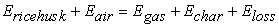(1)

where Erice husk, Eair, Egas, Echar, and Eloss represents energy present in rice husk, air, product gas, unreacted carbon, and energy loss, respectively.

Unequal distribution of air inside the gasifier is responsible for localized pyrolysis, pyrolysis ends at 600 °C. At higher temperatures, thermal cracking reduces the tar content. The total exergy of gas mixture calculated by eq. (2):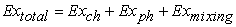(2)

Where Exch, Exph, Exmixing are chemical exergy, physical exergy, and exergy lost due to mixing of gas.

Chemical exergy of a substance is the maximum possible useful work done by the process when the substance brought from environment state to dead state. Chemical exergy of substances obtained from standard chemical exergy tables, specific chemical exergy of substance shown in Table 1.

The specific physical exergy can be calculated 3 using eq. (3):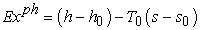(3)

Thus the Total physical exergy can be obtained from: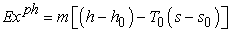(4)

where h, s, h0, and s0 represent specific enthalpy, specific entropy, standard specific enthalpy, and entropy, T0 is atmospheric temperature, and m represents the mass of the component in the stream.

• Figure 1. 5 Reactions Processes in a Downdraft Gasifier 

Specific enthalpy and entropy of gases are calculated as function of temperature given in Table 2 and Table 3.

Exergy lost due to gas mixing 16 calculated from eq. (5):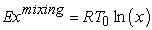(5)

where x is the mole fraction of gas component, R= [8.314 kJ/kmolK]-universal gas constant.

The general exergy balance of a system can be written as 5: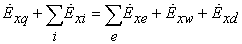(6)

where the subscripts I and e denote the inlet and outlet condition of any component k. The terms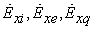and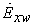refer to inlet and outlet exergy rates, heat transfer exergy rate and exergy due to work, respectively.

In steady state conditions, these quantities can be related as 5: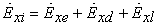(7)

where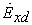represents the exergy destruction, while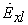represents the waste exergy. The system inefficiencies and exergetic efficiencies for all combined cycle power plants components can be measured from exergy destruction and exergy loss (Table 4) 5, 16, 23. The exergetic efficiency is the ratio of extracted product exergy to the fuel exergy supplied to the component or entire system 5.

2.2. Exergoeconomic Analysis

Exergy costing analysis is a tool used to evaluate the cost of inefficicencies or the costs of individual process streams (including intermediate and final products) but also to improve overall system efficiency and lower life cycle costs of a thermodynamic system. A complete exergoeconomic analysis consists of (1) an exergetic analysis, (2) an economic analysis, and (3) an exergoeconomic evaluation 16, 27. Several studies have focused on exergy costing analyses of power plants and other energy systems have been carried out by researchers 8, 23. The techno-economic feasibility of thermal engineering systems is usually investigated using Life Cycle Cost (LCC) analysis over their expected lifetime. In LCC, the effect of inflation and inetrest rate, maintenance ratio, operating and purchase cost of equipment is used to determine the net cost savings and payback period of initial investment on thermal engineering systems 24. The exergoeconomic approach combines the concepts of exergy and economics to assess the energy system’s cost-effectiveness. The deficiencies of a system can be measured, appraised and, therefore, reduced either during the design or operation stage 5.

2.2.1. Economic Data

The economic data required for the techno-economic study include the cost of electricity per kWh in the selected area, the yearly interest rate for each location, capital cost of acquisition, operation, maintenance cost of the energy scheme as well as its lifetime. For this study, factors such as cost of electricity, yearly interest rate and inflation rate are obtained from the Nigerian Electricity Regulatory Commission (NERC) and National Bureau for Statistics (NBS) respectively. The urban inflation rate increased by 17.59 per cent in August 2021 31 while the electricity tariff in Nigeria as issued by NERC to the Electricity Distribution Companies (DisCos) and Electricity Generation Companies (GenCos) is =N= 24.290 (\$0.059) per kWh (household) and =N= 39.838 (\$0.097) per kWh for businesses 32. Table 5 shows some economic data and assumptions to be used in the course of this study.

2.2.2. Power Plant Costs

Costs are essential and key parameters in power plants. Some of the commonly used metrics 19 are the plant’s capital cost (\$), operational and maintenance (O&M) cost (\$/year) and the cost of electricity (\$/MWh). O&M costs can be divided further, into fixed O&M (FOM), variable O&M (VOM) and fuel cost (FC). This study adopts a hybrid model based and developed from various researches 19, 33, 34, 35.

(a) The total investment cost: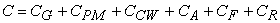(8)

(b) Annual Amount of Electricity (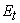) sent out by the power plant in kWh net: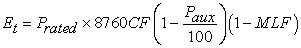(9)

(c) Operating and maintenance cost (O&M):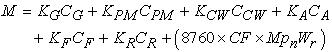(10)

(d) Annual revenue (AR) from the sale of electricity: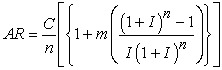(11)

(e) Cost of Fuel (FC):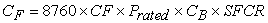(12)

(f) Cost of one kWh of Biomass Gasification electricity generated: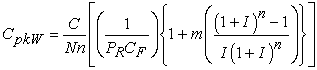(13)

(g) Benefit delivered annually by the Gasification plant: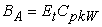(14)

(h) The payback period (PBP) of the gasification project: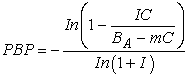(15)

(i) The net present value (NPV) of the gasification project:

(j) The benefit cost ratio (BCR):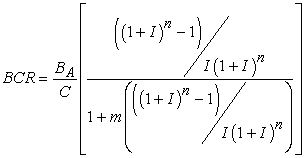(17)

(k) The Internal Rate of Return (IRR):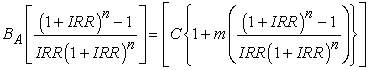(18)

(l) The cost of electricity (COE) or levelized COE (LCOE) is the cost of generating a unit of electricity as shown in Equation (19):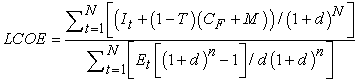(19)

where: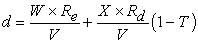(20)

As the produced syngas contains CH4, O2, H2, N2, CO and CO2, some of these gases or components need to be avoided, captured and taken care of, in order to enhance the economic feasibility of the gasification project. Both the CO2 captured and avoided can be mathematically expressed as 19: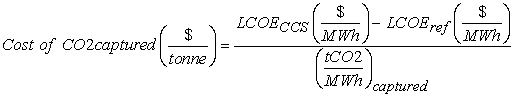(21)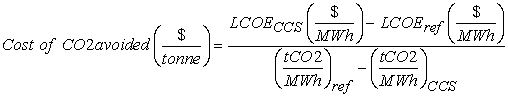(22)

### 3. Results and Discussion

From the steam tables 36, the standard specific enthalpy (h0) and standard specific entropy (s0) can be calculated. In the study area however, the ambient temperature was found to be 37°C, during the period of study; which corresponds to 310 K. This temperature lies between 300 K and 400 K, and not available in the steam tables. Thus, using the linear interpolation technique, the corresponding values of h0 and s0 can be calculated (Table 6).

In order to calculate the molar fraction (x) of each component of the syngas, the typical standard composition of the syngas is used as shown in Table 7 37.

Thus, the molar fractions for the various feedstock loads are calculated using thermodynamic relations 38 as shown in Table 8.

Using the various molar fractions from Table 9, the expression for exergy loss due to gas mixing (Eq. 5), the value of the exergy loss due to gas mixing can be calculated when the mass of the feedstock is 1 kilogram, 1.5 kilograms and 2 kilograms.

Due to the cumbersome nature of the calculations, the total exergies could not be carried out analytically. Therefore, Microsoft Excel was used to compute the values of the total exergies. Table 10 - Table 24 show extracts of the Microsoft Excel computations results for the total exergies of the various gases. The results obtained were used to generate comparative graphs with respect to feedstock loading as shown in Figure 2, Figure 3, Figure 4, Figure 5 and Figure 6.

For the initial load of 1 kg mass of Rice husk feedstock, Table 10 - Table 14 show an extract of some total exergies.

For the load of 1.5 kg mass of Rice husk feedstock, Table 15 - Table 19 show extract of some total exergies.

For the 2 kg mass of Rice husk feedstock, Table 20 - Table 24 show extracts of some total exergies.

• Figure 2. Comparative Graphs of Total Exergies of Methane at Various Load Capacity

Exergy analysis as an important tool to identify and control energy loss during a thermodynamic process, helps determines major contributors to exergy destruction and their readjustment in the process to improve the overall thermodynamic efficiency. For Methane (CH4), the total exergy increases with an increase in the mass of feedstock as shown in Figure 2. However, the situation is being reversed with temperature, where a decrease is noticed with increasing temperature; where values of exergies vary between 1110126 kJ and 1066279 kJ for 2kg; 1037525 kJ and 1004639 kJ for 1.5 kg and finally, 965559.3kJ and 943635.6kJ for a feedstock mass of 1 kg between 690 K and 960 K respectively.

For Nitrogen (N2), as the mass of feedstock increases, so does the total exergy of the process. Furthermore, the total exergy increases with increasing temperature with values of total exergies ranging from 2035.545kJ to 7327.596kJ for a feedstock mass of 1 kg between the temperatures of 690 K and 960K and values ranging between 2943.568 and 10881.64kJ; 4155.17 and 14739.27 kJ for feedstock of 1.5 kg and 2 kg respectively as shown in Figure 3.

In the case of Carbon Monoxide (CO), the total exergy of the process decreases as the mass of feedstock increases as shown in Figure 4; but increases with temperature even though it is almost horizontal if not for the values recorded. Between the temperatures of 690k and 960 K, the total exergies vary between 55789.73 kJ and 66541.6 kJ for a feedstock mass of 2 kg; 109807.3 kJ and 117871.3 kJ for a feedstock mass of 1.5 kg and finally; for an initial feedstock mass of 1 kg, the total exergy values range from 164156.6 kJ to 169532.6 kJ between 690 K and 960 K.

For Carbon Dioxide (CO2), the total exergy of the process increases as the mass of feedstock increases, even though it is almost unnoticeable as shown in Figure 5. So does the total exergy increase with increase in temperature which explains the value of R2 as unity (1). Between the temperatures of 690k and 960 K, the total exergies vary between 480216.2 kJ and 488904.1kJ; 711953.6kJ and 724985.4 kJ; 944034.4kJ and 961410.1kJ for 1kg, 1.5 kg and 2 kg of feedstock respectively.

• Figure 3. Comparative Graphs of Total Exergies of Nitrogen at Various Load Capacity
• Figure 4. Comparative Graphs of Total Exergies of Carbon Monoxide (CO) at Various Masses
• Figure 5. Comparative Graphs of Total Exergies of Carbon Dioxide (CO2) at Various Masses
• Figure 6. Comparative Graphs of Total Exergies of Hydrogen (H2) at Various Load Capacity

Just as in the case of nitrogen, for Hydrogen (H2) too, as the mass of feedstock increases, so does the total exergy of the process. The total exergy increases sharply with increasing temperature as shown in Figure 6 with values of total exergies ranging from 237841.1kJ to 243495.3kJ for a feedstock mass of 1 kg between the temperatures of 690 K and 960 K and values ranging between 239554.9kJ and 248036.1 kJ; 241594.9 kJ and 252903.1 kJ for feedstock of 1.5 kg and 2 kg respectively. These findings are in line with Yueshi et al., 10, who found out that higher preheating temperatures increase the chemical energy of the produced syngas and the two efficiencies.

3.2. Exergoeconomic Analysis Results

Sustainable development not only requires sustainable supply of clean and inexpensive energy source but also efficient use of them. Exergy-based sustainability evaluation is useful to improve efficiency. Table 25 shows a summary of statistical fits for the total exergies per gas and mass of feedstock. From the overall results obtained, the gasification efficiency can be improved by increasing the temperature with an addition of heat in the process. The R2 values of 0.999 to 1 show the true behavior and similarities of the graphs and highlighting the enormous exergy destruction rate in the process. This result strongly compliments those from Mehrpooya et al., 1.

While some components of the syngas contribute towards the energy and heat produced, others need to be avoided, captured and taken care of, in order to enhance the economic feasibility of the gasification project, as earlier mentionned. These are CO2, CO and N2, notably as NOx , bearing in mind their negative impact on the environment as well as the plant performance. Thus, the adoption of a hybrid model, combining the work of Gbeminiyi 35 with the presence of equations expressing parameters: CA (additional costs (labour, repair and maintenance, building services, stores, insurance, and taxes costs)) and KR(fraction of the capital cost of biomass gasification power plant used for the operation and maintenance of accessories and miscellaneous) on one hand. These would not be effective as gases produced are not fully captured. On the other hand, the model presented by Hari 19 mathematically suggested CO2 capture and avoidance. This capture or avoidance would assess their true influence and /or destruction level in the thermodynamic process, as well as contribute towards the financial viability of the gasification plant.

The cost of emissions for CO2, CO and NOx used in this work were adopted from 19 as: 0.024\$/kg, 0.02086 \$/kg and 6.853 \$/kg respectively.

With an increasing awareness of the environmental impacts and practical limitations associated with the traditional fossil energy carriers, many countries aim to increase the efficiency of the processes using energy, while shifting to more sustainable energy sources 20. Exergy –based cost analysis permits a decrease in the production charges of electricity as a significance of an improved exergy presentation of the energy conversion processes.

For the purpose of this study, the exergy results for the 2 kg mass of feedstock were considered. Equations (21) and (22) were adapted and used for evaluation of the economic impact of N2 and eventually NOx too. Table 26 shows the preliminary exergoeconomic analysis results for the gasification plant based on the biomass feedstock and total exergies of gases contained within the syngas. The batch type of gasifier is not suitable because of the time losses incurred during the frequent cleaning, loading and off-loading of gasifier biomass leftover.

### 4. Conclusions

i) The overall results obtained on total exergies of H2, N2, CO, CO2 and CH4, showed that gasification efficiency can be improved by increasing the temperature with an addition of heat in the process.

ii) Methane was found with the highest total exergies where values of exergies were varying between 1110126 kJ and 1066279 kJ for 2kg; 1037525 kJ and 1004639 kJ for 1.5 kg and finally, 965559.3kJ and 943635.6kJ for a feedstock mass of 1 kg between 690 K and 960 K respectively.

iii) The CO2, N2 and NOx capture/ avoidance increases the plant efficiency by producing more electricity thereby increasing the financial potential of the plant.

iv) The total exergies of gases considerably assist in the exergoeconomic analysis of the gasification plant, when the environmental aspect is considered.

### Nomenclature

AR: Annual revenue

BA: Benefit delivered annually

BCR: Benefit cost ratio

C: Total capital investment cost

CpkW: Cost of kWh of electricity generated from the biomass gasification plant

CA: Cost of electricity, accessories, auxiliaries, and miscellaneous

CB: Cost of biomass

CF: Cost of fuel (biomass)

CG: Cost of gasifier or fuel conversion system

CPM: Cost of prime mover

C1: Total capital investment of the project

CCW: Cost of civil work

CR: Additional costs (labour, repair and maintenance, building services, stores, insurance, and taxes costs)

CF: Capacity factor

E: Annual electricity production from the plant

IC: Total project investment cost

I: Real rate of return

IRR: Internal rate of return

KG: Fraction of the capital cost of biomass gasification power plant used for the operation and maintenance of gasifier

KPM: Fraction of the capital cost of biomass gasification power plant used for the operation and maintenance of prime mover (engine)

KCW: Fraction of the capital cost of biomass gasification power plant used for the operation and maintenance of engineering and construction work

KA: Fraction of the capital cost of biomass gasification power plant used for the operation and maintenance of accessories and miscellaneous

KA: Fraction of the capital cost of biomass gasification power plant used for the purchase of fuel and fuel handling

KR: Fraction of the capital cost of biomass gasification power plant used for the labour, repair and maintenance, building services, stores, insurance, and taxes

LCOE: Levelized Cost of Electricity

MEcp: Biomass mass conversion potential, kg/kWh

MLF: Marginal loss factor

MPn: Number of manpower required

m: Percentage of initial investment for annual operating and maintenance costs

N: Annual hour of the plant

n: Life in years of plant

NPV: Net present value

PBP: Payback period of investment

PR: Plant installed capacity

Paux: Percentage power consumption by the auxiliaries

Prated: Power rated or installed output

Re: Nominal cost of debt

Rd: Nominal cost one quity

SFCR: Specific fuel consumption rate

T: Company tax rate

V: Total market value, %

W: Market value on equity, %

Wr: Wage rate for manpower in Nigeria

X: Market value on debt, %.

### Conflicts of Interest

The authors declare that there are no conflicts of interest regarding the publication of the present paper.

### Plagiarism

All works and references consulted have been duly acknowledged. Any omission might have resulted from errors. The authors wish to express their apologies should such a case arise.

### References

Published with license by Science and Education Publishing, Copyright © 2021 Alhaji Bukar Abubakar, Mohammed B. Oumarou, Fasiu A. Oluwole and Sahabo AbubakarThis work is licensed under a Creative Commons Attribution 4.0 International License. To view a copy of this license, visit http://creativecommons.org/licenses/by/4.0/